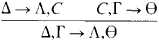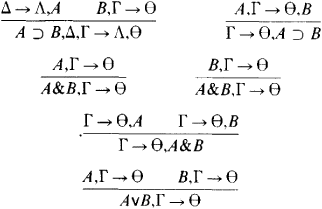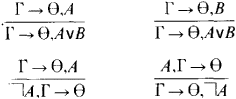# Sequents, Calculus of

The following article is from The Great Soviet Encyclopedia (1979). It might be outdated or ideologically biased.

## Sequents, Calculus of

(or sequential calculus, calculus of methods of inference), a modification of the concept of a logical calculus. In the calculus of sequents, the basic objects that are dealt with are not formulas but sequents—that is, expressions of the form A1,…, Al → B1,…, Bm, where → is analogous to the deducibility symbol and Al…, Al and Bl, …, Bm are arbitrary formulas. The formulas A1 …, Al constitute the antecedent of the sequent, and the formulas B1, …, Bm make up its succedent.

When l, m ≥ 1, the sequent Al …, Al → Bl, … Bm is interpreted as the formula

Al& … &Al⊃ BlV … VBm

where & is the symbol of conjunction, ⊃ of implication, and v of disjunction (seeLOGICAL OPERATIONS). In addition, a sequent with an empty antecedent is interpreted as a truth, a sequent with an empty succedent is interpreted as a falsehood, and, consequently, the sequent →, consisting of just the arrow, is interpreted as a contradiction. All sequents of the form C → C, and only such sequents, are axioms, or initial sequents, in the calculus of sequents.

The rules of inference are divided into structural and logical rules. The former codify permissible changes in the arrangement of the formulas of the antecedent and succedent, and the latter codify the introduction of various logical symbols into the sequents. The structural rules are as follows: refinement, which is the addition of an arbitrary formula to an antecedent or succedent; contraction, which is the deletion of repeating formulas; the transposition of arbitrary formulas in the antecedent or succedent; and the cut rule, that is,Here, the Latin letters denote arbitrary formulas, and the Greek letters denote rows of formulas, which are divided by the commas; the premises of the rule are written above the line, and the conclusion below it. The logical rules of inference have the following form for the sequential classical propositional calculus:If both the structural and logical rules of inference are restricted by the condition that the succedent of each sequent must contain no more than one formula, then we obtain the sequential intuitionistic propositional calculus. This condition is sufficient for the nondeducibility of the law of the excluded middle and of the law of the removal of a double negation in the calculus of sequents. The sequential predicate calculus is obtained by appending to the preceding rules two more pairs of rules for the introduction of the universal and existential quantifiers.

The fundamental result of the German mathematician G. Gentzen consists in the establishment of the possibility of reducing every derivation in the calculus of sequents to a normal form that does not contain applications of the cut rule and that thus in a sense represents a direct derivation. This result has numerous applications. Particularly important are the proofs of the consistency of arithmetic formal systems. These proofs make use of mathematical apparatus that goes beyond the framework of Hilbert’s finitism (seeAXIOMATIC METHOD and METAMATHEMATICS) and thus in a sense bypass the difficulties resulting from K. Gödel’s theorem of the incompleteness of formal arithmetic. Gentzen’s fundamental theorem underlies most algorithms of deducibility for logical and logico-mathematical calculi. This fact accounts for the exceptional importance of the calculus of sequents for the intensively developing research in the field of computerized theorem proving; such research is an important example of the modeling of human mental activity.

### REFERENCES

Gentzen, G. “Issledovaniia logicheskikh vyvodov.” In Matematicheskaia teoriia logicheskogo vyvoda. Moscow, 1967. Pages 9–74. (Translated from German.)
Gentzen, G. “Neprotivorechivost’ chistoi teorii chisel.” Ibid., pp. 77–153. (Translated from German.)
Gentzen, G. “Novoe izlozhenie dokazatel’stva neprotivorechivosti dlia chistoi teorii chisel.” Ibid., pp. 154–90.
Curry, H. B. Osnovaniia matematicheskoi logiki. Moscow, 1969. Chapters 5C, 6B, 7B, and 8B. (Translated from English.)
Algorifm mashinnogo poiska estestvennogo logicheskogo vyvoda v ischisle-nii vyskazyvanii. Moscow-Leningrad, 1965.